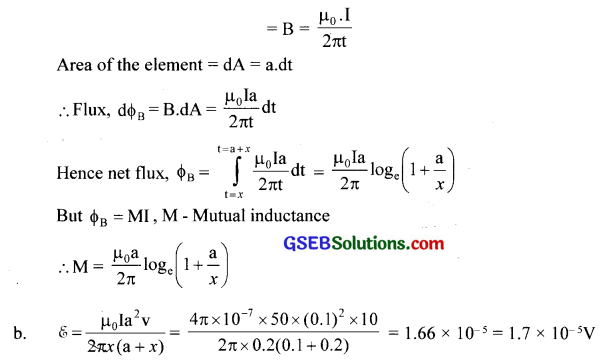# GSEB Solutions Class 12 Physics Chapter 6 Electromagnetic Induction

Gujarat Board GSEB Textbook Solutions Class 12 Physics Chapter 6 Electromagnetic Induction Textbook Questions and Answers, Additional Important Questions, Notes Pdf.

## Gujarat Board Textbook Solutions Class 12 Physics Chapter 6 Electromagnetic Induction

### GSEB Class 12 Physics Electromagnetic Induction Text Book Questions and AnswersQuestion 1.
Predict the direction of induced current in the situations described by the following figures (a) to (f ).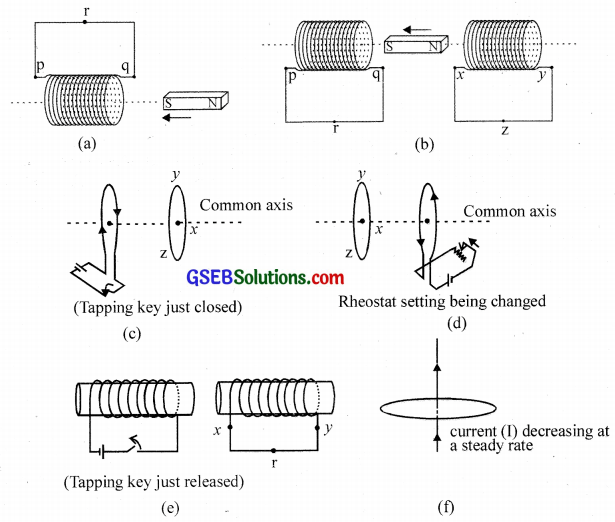Solution:
(a) According to Lenz’s law, the end of the coil facing the S-pole of the magnet coil becomes an S-pole so as to repel the approaching magnet. For this to happen, the current in the coil will flow in the direction QRPQ.

(b) For the coil on left: For the reason given in (a), the current in the left coil will flow in the direction of PRQP.
For the coil on right: According to Lenz’s law, the end of the coil facing the N-pole of the magnet will become S-pole so as to attract the magnet moving away from it. For this to happen, the current in the coil will flow in the direction YZXY.

(c) When the tapping key is just closed, the current flow’s in the coil and hence magnetic flux linked with it starts growing. According to Lenz’s law, the induced current in the adjoining coil should set up in a direction so as to oppose the growth of magnetic flux linked with the coil. It will happen so if the direction of the magnetic field produced by the induced current in the adjoining coil is from right to left i.e. the induced current is produced in the direction YZX.

(d) Due to change in rheostat setting (resistance decreased), the current in the coil and hence magnetic flux linked with it, will start increasing. According to Lenz’s law, the induced current in the adjoining coil should set up in a direction. so as to oppose the increase in magnetic flux linked with the coil. It will happen. So, if the direction of the magnetic field produced by the induced current in the adjoining coil is from right to left i.e. the induced current is produced in the direction ZYX.

(e) Before releasing the tapping keg, the right end of the core is N-pole and the magnetic field linked with the coil is from left to right. As the tapping keg is released, the magnetic field linked with the coil will start collapsing. According to Lenz’s Law, the induced current in the adjoining coil should set up in a direction so as to reinforce the collapsing magnetic field. It will happen so if the direction of the magnetic field produced by the induced current in the adjoining coil is from left to right i.e. the induced current is produced in the direction XRY.

(f) The current flowing through the straight conductor produces a magnetic field in the plane of the coil. Since no magnetic flux is linked with the coil, no induced current will be set up in the coil.

Question 2.
Use Lenz’s law to determine the direction of induced current in the situations described by the following figures.(a) A wire of irregular shape turning into a circular shape;
(b) A circular loop being deformed into a narrow straight wire.
Solution:
(a) By right hand thumb rule, the current direction is along adcba.
(b) Here current direction is opposite to that in fig. (a) i.e., along a’d’c’b’a’.Question 3.
A long solenoid with 15 turns per cm has a small loop area 2.0 cm2 placed inside the solenoid normal to its axis. If the current carried by the solenoid changes steadily from 2.0 A to 4.0 A in 0.1 s, what is the induced emf in the loop while the current is changing?
Solution:Question 4.
A rectangular wire loop of sides 8 cm and 2 cm with a small cut is moving out of a region of the uniform magnetic field of magnitude 0.3 T directed normal to the loop. What is the emf developed across the cut if the velocity of the loop is 1cm s-1 in a direction normal to the (i) longer side, (ii) shorter side of the loop? For how long does the induced voltage last in each case?
Solution:
(i) Direction of motion normal to longer side: Length of the longer side,
1 = 8 cm = 8 x 10-2 m
∴ e = BlV = 0.3 x 8 x 10-2 ×10-2 = 0.24 x 13-3V = 0.24 mV
The.e.m.f. will last in the loop, till it does not get out of the magnetic field i.e. for the time the loop takes to travel a distance equal to the length of the shorter arm. If t is the time for which the e.m.f lasts in the loop, then
$$t=\frac { length\quad of\quad the\quad shorter\quad arm }{ V } =\frac { 2\times { 10 }^{ -2 } }{ { 10 }^{ 2 } } =2S$$

(ii) Direction of motion normal to the shorter side:
Length of the shorter side, 1 = 2 cm = 2 x 10-2m
∴ e = Blv = 0.3 x 2 x 102 x 10-2 = 0.06mV
The time for which the e.m.f. lasts in the loop,
$$t=\frac { length\quad of\quad the\quad longer\quad arm }{ V } =\frac { 8\times { 10 }^{ -2 } }{ { 10 }^{ -4 } } =8S$$Question 5.
A 1.0 m long metallic rod is rotated with an angular frequency of 400 rad s-1 about an axis normal to the rod passing through its one end. The other end of the rod is in contact with a circular metallic ring. A constant and uniform magnetic field of 0.5 T parallel to the axis exists everywhere. Calculate the emf developed between the centre and the ring.
Solution:
$$\mathscr{E}$$ = $$\frac { Blv }{ 2 }$$ = $$\frac{\mathrm{B} l^{2} \cdot \omega}{2}$$ [∴ v = lω] = $$\frac { 0.5×1×1×400 }{ 2 }$$ = 100 V

Question 6.
A circular coil of radius 8.0 cm and 20 turns is rotated about its vertical diameter with an angular speed of 50 rad s-1 in a uniform horizontal magnetic field of magnitude 3.0 x 10-2 T. Obtain the maximum and average emf induced in the coil. If the coil forms a closed loop of resistance 10 Ω, calculate the maximum value of current in the coil. Calculate the average power loss due to Joule heating. Where does this power come from?
Solution:
For a coil rotating in a magnetic field, $$\mathscr{E}$$ = – BANωinωt
$$\mathscr{E}$$ = – BANω (numerically)
Here B = 3 x 10-2 T, A= π2= π x (8 x 10-2)2
N = 20, ω = 50 rad s-1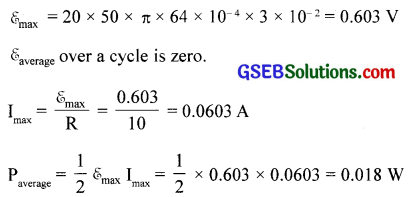The Source of power loss is the external rotor which provides the necessary torque.

Question 7.
A horizontal straight wire 10 m long extending from east to west is falling with a speed of 5.0 m s-1, at right angles to the horizontal component of the earth’s magnetic field, 0. 30 x 10-4 Wb m-2.
(a) What is the instantaneous value of the emf induced in the wire?
(b) What is the direction of the emf?
(c) Which end of the wire is at the higher electrical potential?
Solution:
Here, length of the wire, 1 = 10m;
Velocity of the wire, V = 5.0 ms1
Horizontal component of earth’s magnetic field,
BH = 0.30 x 10-4 Wb m-2
(a) Now,e = BHIv = 0.30 x l0-4x 10 x 5.0 = 1.5 x 10-3 V
(b) The induced e.m.f. will be set up from west to east end.
(c) The eastern end will be at higher potential.Question 8.
Current in a circuit falls from 5.0 A to 0.0 A in 0.1s. If an average emf of 200 V is induced, give an estimate of the self-inductance of the circuit.
Solution:Question 9.
A pair of adjacent coils has a mutual inductance of 1.5 H. If the current in one coil changes from 0 to 20 A in 0.5 s, what is the change of flux linkage with the other coil?
Solution:
Hers, M = 1.5H; dl = 20-0 = 20A;
dt = 0.5 s
Now φ = MI
Or dφ= Mdl=1.5 x 20 = 30Wb.

Question 10.
A jet plane is traveling towards the west at a speed of 1800 km/h. What is the voltage difference developed between the ends of the wing having a span of 25 m, if the earth’s magnetic field at the location has a magnitude of 5 x 10-4 T and the dip angle is 30°?
Solution:
Here, v = 1,800 Km h-4 = 1,800 x [1,000 m] x [60 x 60 s]-1 = 500 ms-1
Earth’s magnetic field, B = 5.0 x 10-4 t;
angle of dip, 5 = 30°; length of the wing = 25 m
Now, Bv = B sin5 = 5.0x 10-4 sin 30° = 2.5×10-4T
The vertical component [Bv] of the earth’s field is normal to both the wings and the direction of motion. Therefore, induced e.m.f. produced,
e = Bv x l x V = 2.5 x 500 = 3.125V.

Question 11.
Suppose the loop in Exercise.4 is stationary but the current feeding the electromagnet that produces the magnetic field is gradually reduced so that the field decreases from its initial value of 0.3 T at the rate of 0.02 T s-1. If the cut is joined and the loop has a resistance of 1.6 Ω, how much power is dissipated by the loop as heat? What is the source of this power?
Solution:
$$\mathscr{E}$$ = $$\frac{\mathrm{d} \phi_{\mathrm{B}}}{\mathrm{dt}}$$ = A.$$\frac { dB }{ dt }$$
= 8 x 2 x 10-4 x 0.02 = 3.2 x 10-5 V
Induced current, I = $$\frac{\mathscr{E}}{\mathrm{R}}$$
= $$\frac{3.2 \times 10^{-5}}{1.6}$$
= 2 x 10-5 A
Power loss = P = $$\mathscr{E}$$I= 3.2 x 10-5 x 2 x 10-5
= 6.4 x 10-10 W
Source of this power is the external agency which brings the change in the magnetic field.Question 12.
A square loop of side 12 cm with its sides parallel to X and Y axes is moved with a velocity of 8 cm s-1 in the positive x-direction in an environment containing a magnetic field in the positive z-direction. The field is neither uniform in space nor constant in time. It has a gradient of 10-3 T cm-1 along the negative x-direction (that is it increases by 10-3 T cm-1 as one move in the negative x-direction), and it is decreasing in time at the rate of 10-3 T s-1. Determine the direction and magnitude of the induced current in the loop if its resistance is 4.50 m Ω.
Solution:
Rate of change of flux due to time variation in B = 144 x 10-4m2 x 10-3 Ts-1 = 1.44 x 10-5 Wb/s
Rate of change of flux due to motion of the loop in nonuniform
B = 144 x 10-4 m2 x 10-3 Tcm-1 x 8 cm/s = 11.52 x 10-5 Wb/s
Net change in flux = $$\mathscr{E}$$ = (1.44 + 11.52) x 10-5 = 12.96 x 10-5 V
Current I = $$\frac{12.96 \times 10^{-5}}{4.5 \times 10^{-3}}$$ = 2.88 x 10-2 A
The direction of the induced current is such that it increases the magnetic flux linked with the loop in the positive direction.

Question 13.
It is desired to measure the magnitude of the field between the poles of a powerful loudspeaker magnet. A small flat search coil of area 2 cm2 with 25 closely wound turns, is positioned normal to the field direction and then quickly snatched out of the field region. Equivalently, one can give it a quick 90° turn to bring its plane parallel to the field direction. The total charge flew in the coil (measured by a ballistic galvanometer connected to the coil) is 7.5mC. The combined resistance of the coil and the galvanometer is 0.50 Ω. Estimate the field strength of the magnet.
Solution: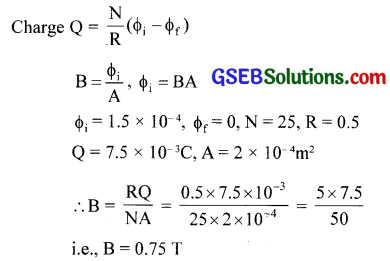Question 14.
An air-cored solenoid with a length of 30 cm, area of cross-section 25 cm2, and a number of turns 500, carries a current of 2.5 A. The current is suddenly switched off in a brief time of 10-3 s. How much is the average back emf induced across the ends of the open switch in the circuit? Ignore the variation in the magnetic field near the ends of the solenoid.
Solution:### GSEB Class 12 Physics Electromagnetic Induction Additional Important Questions and Answers

Question 1.
A solenoid with a large number of turns is in a closed circuit (See Fig.E.3) and a short bar magnet is dropped through each with its length along the axis. State the acceleration of the falling magnet when it is:
(a) Well above A
(b) At the end A
(c) At the middle
(d) At the end B
(e) Far away, down, from B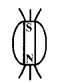(a) Acceleration due to gravity (g)
(b) Less than g by Lenz’s law.
(c) Same as in (a)
(d) Less than g, by Lenz’s law.
(e) Free from induction and hence a = gQuestion 2.
A rectangular closed loop moves horizontally in a uniform magnetic field.
(i) Will there be any induced current in the loop if the loop is completely in the magnetic field?
(ii) Will there be any induced current in the loop if the loop is partially out of the magnetic field?
(iii) Will the induced current in the loop remain if the loop is stationary and the magnetic field changes with time?
(i) No. There is no change of flux and hence no induced e.m.f
(ii) Yes. Because there is no change of magnetic flux.
(iii) Yes. The time-varying flux will induce an e.m.f. or current in the loop.

Question 3.
(a) How is the flux linkage through a coil related to current?
(b) What is the constant of proportionality that appears in the above relation?
(c) Define the constant of proportionality.
(d) Give its dimensions and S. I. unit.
(a) For a current-carrying coil, flux is proportional to current, i.e., ΦB ∝ I or ΦB = LI
(b) Coefficient of self-induction (L).
(c) Self-inductance of a coil is the magnetic flux linked with the coil when unit current flows through it.
(d) Dimension of L = [ ML2 T-2 L2 ] and S.I. unit is henry

Question 4.
The induced emf is sometimes called back emf Why?
It is because induced emf opposes the applied voltage.Question 5.
Why is spark produced in the switch of a fan when it is switched off?
Due to the sudden break, a largely induced emf is set up across the gap in the switch. Hence the reason.

Question 6.
Fig. E. l shows magnet coil experiment of electromagnetic induction.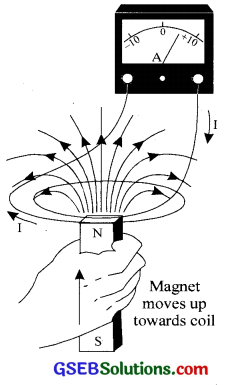What happens when
(a) i. The number of turns of the coil is increased.
ii. The strength of the magnet is increased.
iii. The speed of motion of the magnet is increased.
(b) An induced e.m.f. has no direction of its own. Why?
(a) i. Induced e.m.f increases
ii. induced e.m.f increases
iii. induced e.m.f increases

(b) The direction of induced e.m.f or current is such as to oppose the cause produced it. In this experiment, if the direction of motion of the magnet reverses, the e.m.f’s direction also reversed. Hence an induced e.m.f has no direction of its own.Question 7.
Take a strong cylindrical electromagnet connected to the AC source and place a light metallic disc at the top of it. When the current is switched ‘on the disc is thrown up in the air.
(a) Why does the disc go up?
(b) How is the repulsive force produced?
(a) Induced currents are produced in the disc which acts in such a manner that it opposes the increase of magnetic flux through it. As a result, the disc is thrown up.

(b) When the circuit is switched on, the current start growing through the solenoid. As the current grows, the magnetic lines along the axis of the solenoid and hence magnetic flux through the disc also increases from zero to some finite value. Induced currents are produced in the disc which opposes the increase of magnetic flux through it. Hence the disc is thrown up.

Question 8.
A short bar magnet is dropped through a coil of wire of similar length (Fig.E. 2). Which one of the graphs below shows best how the current through the coil varies with time?D. Because graph D shows best how the current flows through the coil varies with time. It depends on the nature of cause that produces it.

Question 9.
When a metallic block moves in a magnetic field induced currents are developed in the body of the block.
(a) What is this type of current called?
(b) Which law gives the direction of such currents?
(c) What is the strength of the induced current?
(a) Eddy currents
(b) Lenz’s law
(c) Since the metallic block has very small resistance, strength of eddy current will be very large.

Question 10.(a) Which law is demonstrated in the above figures?
(b) State the law.
(c) Explain the action that takes place in the above figures.
(a) Lenz’s law.

(b) The direction of the induced e.m.f. is given by Lenz’s law. The law states that the direction of the induced e.m.f. or current is such as to oppose the cause that produced it.
i.e., $$\mathscr{E}$$ ∝ $$\frac{-\mathrm{d} \phi_{\mathrm{B}}}{\mathrm{dt}}$$, $$\mathscr{E}$$ = $$\frac{-\mathrm{d} \phi_{\mathrm{B}}}{\mathrm{dt}}$$, the constant of proportionality is unity.

(c) In fig E.4a, when the N-pole is introduced in the coil, induced current flows so that the current in the coil is anticlockwise as seen from the left. In fig E.4b, as the magnet is taken away, the induced current flowing clockwise at the left end produces a south pole opposing the motion of the North pole away.

Question 11.
When the key is closed, a spark is generated between the ends of the rods (see Fig. E.6).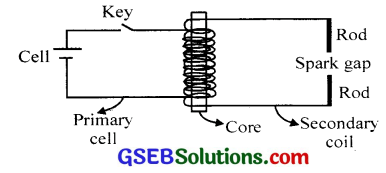(a) Explain the cause of the spark.
(b) Explain why a spark is also produced when the key is opened.
(c) Why are no sparks produced when the key is left closed?
(a) The induced e.m.f is secondary
(b) During decay actually a large e.m.f should be induced in the secondary
(c) No variation of flux takes placeQuestion 12.
You are given a solenoid of length ‘l’, number of turns per unit length ‘n’, and area of cross-section A. When a current ‘I’ passes through it,
(a) What is the total magnetic flux through the solenoid?
(b) What is the self-inductance of the solenoid?
(c) Flow does the value of self-inductance get affected if some material of high relative permeability is filled inside the
(a) μ0nlAN or $$\frac{\mu_{0} \mathrm{n}^{2} \mathrm{IA}}{l}$$
(b) L = $$\frac{\mu_{0} \mathrm{~N}^{2} \mathrm{~A}}{l}$$
(c) It increases p.r times that given in (b)

Question 13.
The figure shows a metal rod PQ resting on the smooth rails AB and positioned between the poles of a permanent magnet. The rails, the rod, and the magnetic field are in three mutually perpendicular directions. A galvanometer G connects the rails through a switch K. Length of the rod = 15 cm, B = 0.50 T resistance of the closed-loop containing the rod = 9.0 m Ω. Assume the field to be uniform.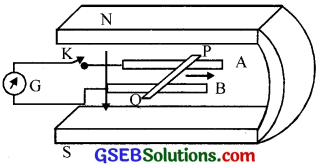(a) Suppose K is open and the rod is moved with a speed of 12 cm s-1 in the direction shown. Give the polarity and magnitude of the induced emf.

(b) Is there an excess charge built up at the ends of the rods when K is open? What if K is closed? With K open and the rod moving uniformly, there is no net force on the electrons in the rod PQ even though they do experience a magnetic force due to the motion of the rod. Explain.

(c) With K open and the rod moving uniformly, there is no net force on the electrons in the rod PQ even though they do experience a magnetic force due to the motion of the rod. Explain.

(d) What is the retarding force on the rod when K is closed?

(e) How much power is required (by an external agent) to keep the rod moving at the same speed (= 12 cm s-1) when K is closed? How much power is required when K is open?

(f) How much power is dissipated as heat in the closed-circuit? What is the source of this power?

(g) What is the induced emf in the moving rod if the magnetic field is parallel to the rails instead of being perpendicular?
Solution:
(a) $$\mathscr{E}$$ = Blv = 0.5 x 15 x 10-2 x 12 x 10-2 = 9.0 mV
P is the positive end and Q the negative end. [The conductor moves towards this end)

(b) Yes. On closing K, current flows but the excess charge is maintained as long as the change continues.

(c) Magnetic force on the charge is cancelled by the electric force set up due to the excess charge of opposite signs at the ends of the rod.

(d) F = BIl = 75 x 10-3 N

(e) Power = F.v = 9 x 10-3 W. No power is expended when K is open (no current)

(f) I2 R = 9.0 x 10-3 W. Source of power is supplied by the external agent.

(g) Zero. No change of flux.Question 14.
(a) Obtain an expression for the mutual inductance between a long straight wire and a square loop of side an as shown in the figure.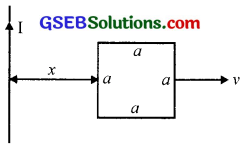(b) Now assume that the straight wire carries a current of 50 A and the loop is moved to the right with a constant velocity, v = 10 m/s. Calculate the induced emf in the loop at the instant when x = 0.2 m. Take a = 0.1 m and assume that the loop has a large resistance.Solution:
(a) Consider a small rectangular element of the square, of thickness dt at a distance ‘t’ from the conductor carrying a current I.
Magnetic field experienced by this element